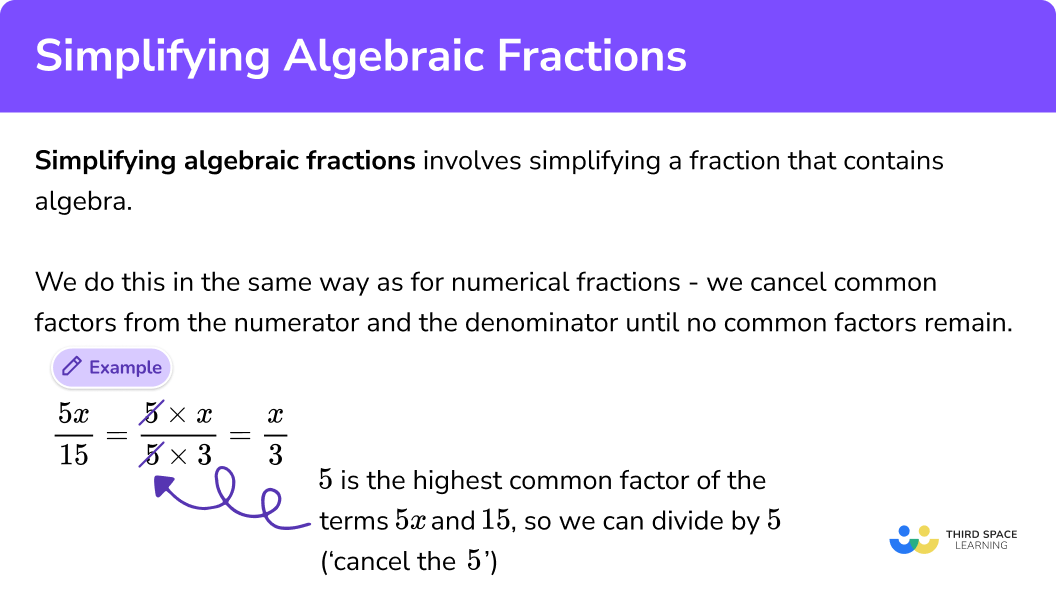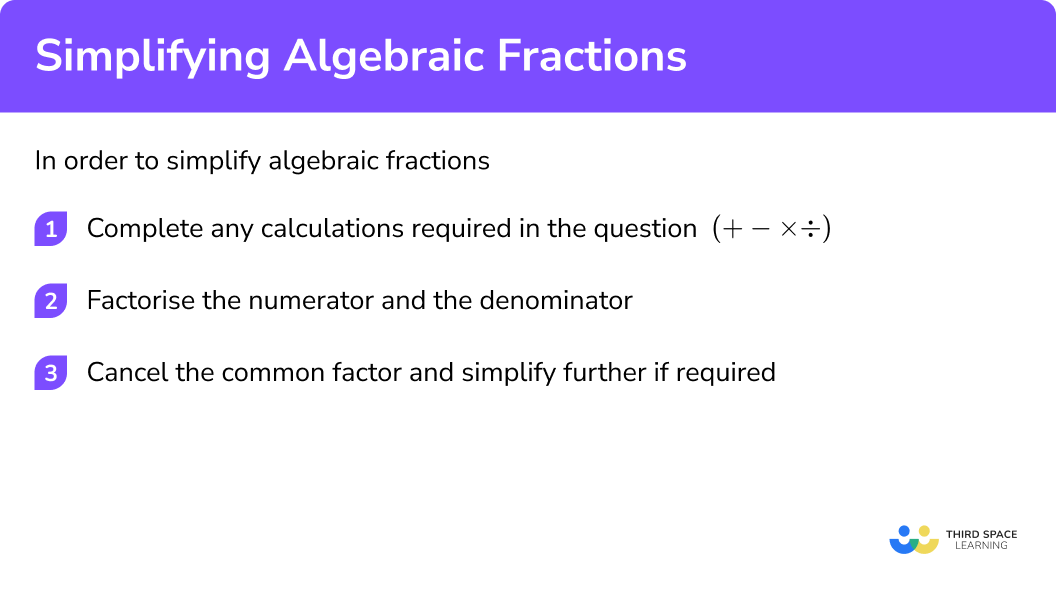# Simplifying Algebraic Fractions

Here we will learn about simplifying algebraic fractions, including different powers of x, quadratics, and the difference of two squares.

There are also simplifying algebraic fractions worksheets based on Edexcel, AQA and OCR exam questions, along with further guidance on where to go next if you’re still stuck.

## What is simplifying algebraic fractions?

Simplifying algebraic fractions is simplifying a fraction that contains algebra so that the numerator and the denominator do not contain any common factors.

To do this, we need to be able to find common factors between the numerator and the denominator which can be cancelled down.

In order to simplify algebraic fractions, you must be confident with calculating with fractions.

If we want to express \frac{a}{b}+\frac{c}{d} or \frac{a}{b} - \frac{c}{d} as a single fraction, we need to find a common denominator and then add or subtract the resultant fractions.

The general form when adding fractions is:

The general form for subtracting fractions is:

E.g.
Calculate \frac{2}{5}+\frac{1}{4}

So if a = 2, b = 5, c = 1 and d = 4 , we can therefore say that

\frac{2}{5}+\frac{1}{4}=\frac{8}{20}+\frac{5}{20}=\frac{8+5}{20}=\frac{13}{20}

Below is a visual representation of this problem,

By converting the fractions so that they have a common denominator (in this case 20 ), we divide the fractions into smaller parts, then total those parts, all by using knowledge of equivalent fractions.

We can use this to simplify algebraic expressions:

E.g.
Let’s look at \frac{5x}{15}

Here, the terms 5x and 15 share a common factor of 5 .

If we wrote this out as two products, we would get \frac{5\times{x}}{5\times{3}} .

We can simplify this by cancelling the common factor of 5 from the numerator and the denominator of the fraction \frac{5\times{x}}{5\times{3}}=\frac{x}{3} .

So by finding the highest common factor of the numerator and the denominator, we can show that the fraction \frac{5x}{15} can be expressed as as the more simple algebraic fraction \frac{x}{3} .

We can use knowledge of simplifying algebraic fractions to solve equations that include algebraic fractions. For more information on this, see the lesson on algebraic fractions.

Step-by-step guide: Algebraic fractions

### What is simplifying algebraic fractions?## Simplify algebraic products and quotients

Sometimes algebraic fractions can be simplified by using the index laws. Here is an example where a product and a quotient can be simplified.

E.g.

Simplify

\frac{4x^5 \times 3x^2}{2x^3}

This can be simplified by first considering the numerator can be simplified using the product law of indices.

\frac{4x^5 \times 3x^2}{2x^3}=\frac{12x^7}{2x^3}

Then the fraction can be further simplified by using the quotient law of indices.

\frac{12x^7}{2x^3}=6x^4

## How to simplify algebraic fractions

In order to simplify algebraic fractions:

1. Complete any calculations required in the question (+ − \times\div) .
2. Factorise the numerator and the denominator.
3. Cancel the common factor and simplify further if required.

### Explain how to simplify algebraic fractions## Simplifying algebraic fractions examples

### Example 1: Multiplying algebraic fractions

Simplify the algebraic expression

\frac{5}{x+2}\times\frac{x+2}{10}

1. Complete any calculations required in the question (+−\times\div) .

\frac{5}{x+2}\times\frac{x+2}{10}=\frac{5x+10}{10x+20}

2Factorise the numerator and the denominator.

\frac{5x+10}{10x+20}=\frac{5(x+2)}{10(x+2)}

3Cancel the common factor and simplify further if required.

\frac{5(x+2)}{10(x+2)}=\frac{5}{10}=\frac{1}{2}

### Example 2: Adding algebraic fractions

Simplify the algebraic expression

\frac{10}{6+2x}+\frac{3}{x+3}

Complete any calculations required in the question (+−\times\div) .

Factorise the numerator and the denominator.

Cancel the common factor and simplify further if required.

### Example 3: Linear ÷ linear

Simplify the algebraic fraction

\frac{6x+12}{10x+20}

Complete any calculations required in the question (+−\times\div) .

Factorise the numerator and the denominator.

Cancel the common factor and simplify further if required.

### Example 4: Linear ÷ quadratic

Simplify the algebraic fraction

\frac{2x+6}{x^{2}+11x+24}

Complete any calculations required in the question (+−\times\div) .

Factorise the numerator and the denominator.

Cancel the common factor and simplify further if required.

Simplify the algebraic fraction

\frac{x^{2}-3x-10}{x^{2}+2x-35}

Complete any calculations required in the question (+−\times\div) .

Factorise the numerator and the denominator.

Cancel the common factor and simplify further if required.

Simplify the algebraic fraction

\frac{x^{2}+15x+56}{x^{2}-64}

Complete any calculations required in the question (+−\times\div) .

Factorise the numerator and the denominator.

Cancel the common factor and simplify further if required.

### Common misconceptions

• Ignoring the denominators

When given an equation including an algebraic fraction, the denominators are ignored so the question is answered incorrectly.

When adding two fractions, the denominator must be the same. A common misconception for adding two fractions is to add the numerators and the denominators together because this method is emphasised when looking at multiplying fractions.

• Cancelling terms incorrectly

Seeing the same term on the numerator and denominator can result in the misconception that they can both be cancelled.

• Expanding double brackets incorrectly

When expanding double brackets, each term in the bracket needs to be multiplied by each term in the other bracket. By not treating the numerator as a single expression, mistakes are made when multiplying brackets together.

• Collecting un-like terms

Simple algebraic expressions such as 5x + 4 can be incorrectly simplified to get 9x as the x is ignored; the assumption is that 5x + 4 is the same as (5 + 4)x which is not correct.

• Factorising into a single bracket incorrectly

When factorising into a single bracket, the incorrect method occurs when the bracket is simply placed between the number and the x of the first term, then around the last term in the expression.

E.g.

Factorising 3x + 12 incorrectly, we would get 3(x + 12)

Here the bracket is placed between the 3 and the x , and the 12 has not been factorised at all. When factorising, the common factor is placed on the outside of the bracket, and the factor pair that generates the term is placed on the inside, so for the example given 3x + 12 = 3(x + 4) .

### Practice simplifying algebraic fractions questions

1. Simplify the fraction

\frac{2x+6}{x+1}\div{\frac{x+3}{x+5}}

\frac{2}{\frac{x+1}{x+5}}\frac{8x+5}{2x+3}\frac{2(x+5)}{x+1}\frac{2}{3}\begin{aligned} &\frac{2x+6}{x+1}\div{\frac{x+3}{x+5}}\\\\ &=\frac{2x+6}{x+1}\times{\frac{x+5}{x+3}} \\\\ &=\frac{2(x+3)}{x+1}\times{\frac{x+5}{x+3}}\\\\ &=\frac{2(x+3)(x+5)}{(x+1)(x+3)}\\\\ &=\frac{2(x+5)}{x+1} \end{aligned}

2. Simplify the fraction

\frac{3}{4x^{2}}-\frac{1}{2x}

\frac{3-2x}{4x^{2}}\frac{2}{2x}\frac{2}{4x^{2}-2x}\frac{3-2x}{4x^{2}}\begin{aligned} &\frac{3}{4x^{2}}-\frac{1}{2x}=\frac{3}{4x^{2}}-\frac{2x}{4x^{2}}\\\\ &=\frac{3-2x}{4x^{2}} \end{aligned}

3. Simplify the fraction

\frac{10x+12}{15x+18}

1\frac{22x}{33x}\frac{2}{3}\frac{2(x+12)}{3(x+18)}\frac{10x+12}{15x+18}=\frac{2(5x+6)}{3(5x+6)}=\frac{2}{3}

4. Simplify the algebraic fraction

\frac{9x-27}{x^{2}+4x-21}

\frac{9}{(x-7)}\frac{3(3x-9)}{(x+7)(x-3)}\frac{9}{x+7}\frac{-18}{x-17}\begin{aligned} &\frac{9x-27}{x^{2}+4x-21}=\frac{9(x-3)}{(x+7)(x-3)}\\\\ &=\frac{9}{x+7} \end{aligned}

5. Simplify the algebraic fraction

\frac{x^{2}+2x-24}{x^{2}+x-30}

\frac{x-4}{x-5}\frac{x-22}{x-31}\frac{x+4}{x+5}\frac{x+8}{x+10}\begin{aligned} &\frac{x^{2}+2x-24}{x^{2}+x-30}=\frac{(x+6)(x-4)}{(x+6)(x-5)}\\\\ &=\frac{x-4}{x-5} \end{aligned}

6. Simplify the algebraic fraction

\frac{x^{2}+6x-7}{49-x^{2}}

\frac{x+1}{x+7}\frac{x-1}{7-x}\frac{x-1}{48}\frac{x-1}{x-7}\begin{aligned} &\frac{x^{2}+6x-7}{49-x^{2}}=\frac{(x+7)(x-1)}{(7+x)(7-x)}\\\\ &=\frac{(x+7)(x-1)}{(x+7)(7-x)}\\\\ &=\frac{x-1}{7-x} \end{aligned}

### Simplifying algebraic fractions GCSE questions

1. Simplify the algebraic fraction

\frac{x^{2}-9x+20}{5x-20}

Write your solution in the form \frac{ax+b}{c} where a, b, and c are integers.

(3 marks)

Numerator is factorised: (x-5)(x-4)

(1)

Denominator is factorised: 5(x-4)

(1)

Fraction is simplified: \frac{x-5}{5}

(1)

2. (a)  Justin is simplifying the algebraic fraction \frac{x^{2}-4}{x+2}

His work is written below.Is Justin correct?

(b)  Show that the fraction \frac{9x^{2}-16}{3x-4} can be simplified to 3x+4 .

(5 marks)

(a)

Justin has the correct answer but he has not shown the correct understanding

(1)

He should have factorised the numerator to get (x+2)(x-2)

(1)

He can then cancel the common factor of (x+2) , leaving the solution x-2

(1)

(b)

9x^2-16=(3x+4)(3x-4)

(1)

\frac{(3x+4)(3x-4)}{(3x-4)}=3x+4

(1)

3. Simplify fully

\frac{2x+1}{2x+10}\div{\frac{3+6x}{x+5}}

(4 marks)

\frac{2x+1}{2x+10}\div{\frac{3+6x}{x+5}}=\frac{2x+1}{2x+10}\times{\frac{x+5}{3+6x}}

(1)

=\frac{2x+1}{2(x+5)}\times{\frac{x+5}{3(1+2x)}}

(1)

=\frac{(2x+1)(x+5)}{6(x+5)(2x+1)}

(1)

=\frac{1}{6}

(1)

## Learning checklist

You have now learned how to:

• Simplify and manipulate algebraic expressions (including those involving surds {and algebraic fractions}) by:
• Factorising quadratic expressions of the form x2 + bx + c, including the difference of 2 squares; {factorising quadratic expressions of the form ax2 + bx + c}
• Simplifying expressions involving sums, products and powers, including the laws of indices

## Still stuck?

Prepare your KS4 students for maths GCSEs success with Third Space Learning. Weekly online one to one GCSE maths revision lessons delivered by expert maths tutors.

Find out more about our GCSE maths tuition programme.